## Basic goodness-of-fit plots

### Plots of the independent variable (IDV)

dv_vs_idv(xpdb_ex_pk)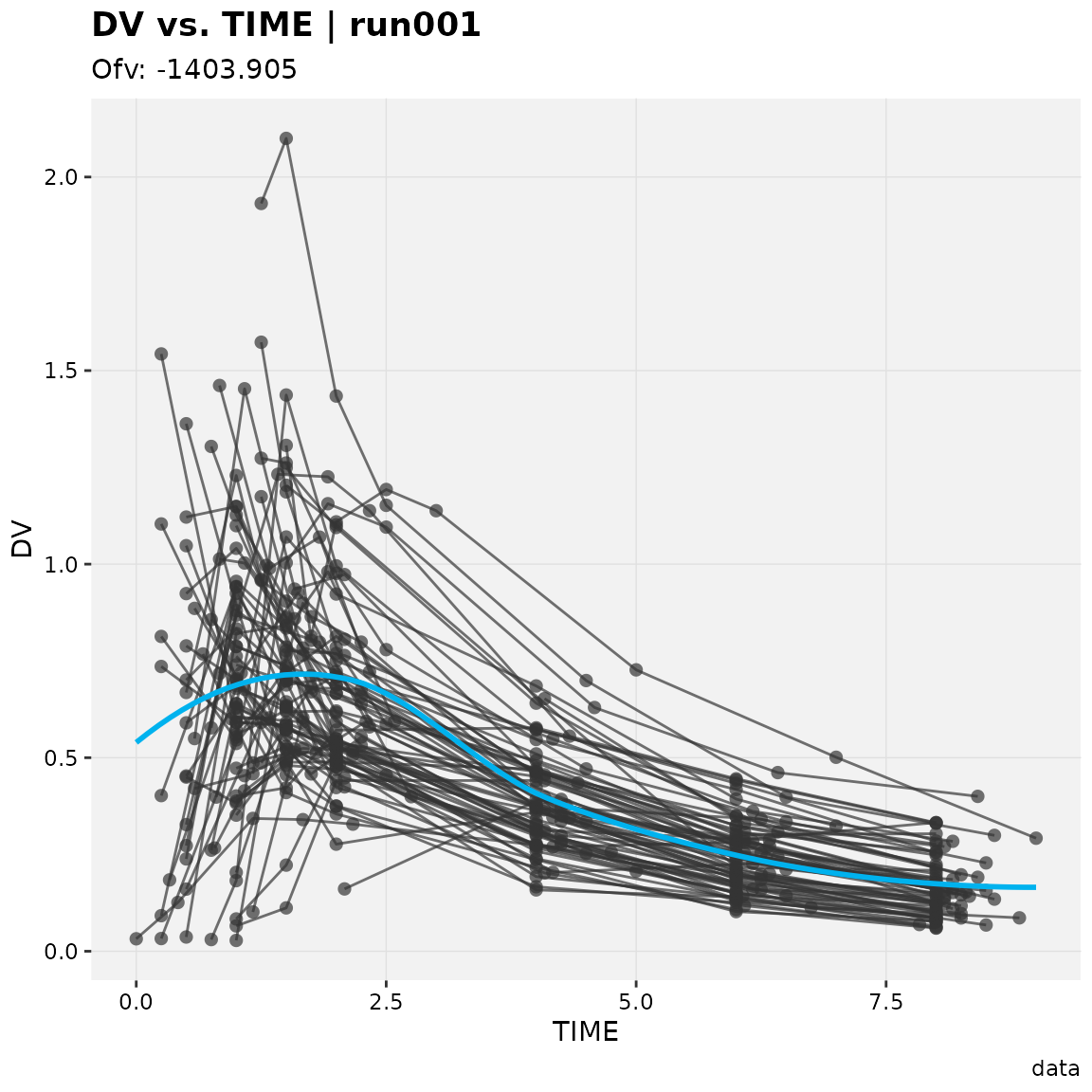ipred_vs_idv(xpdb_ex_pk)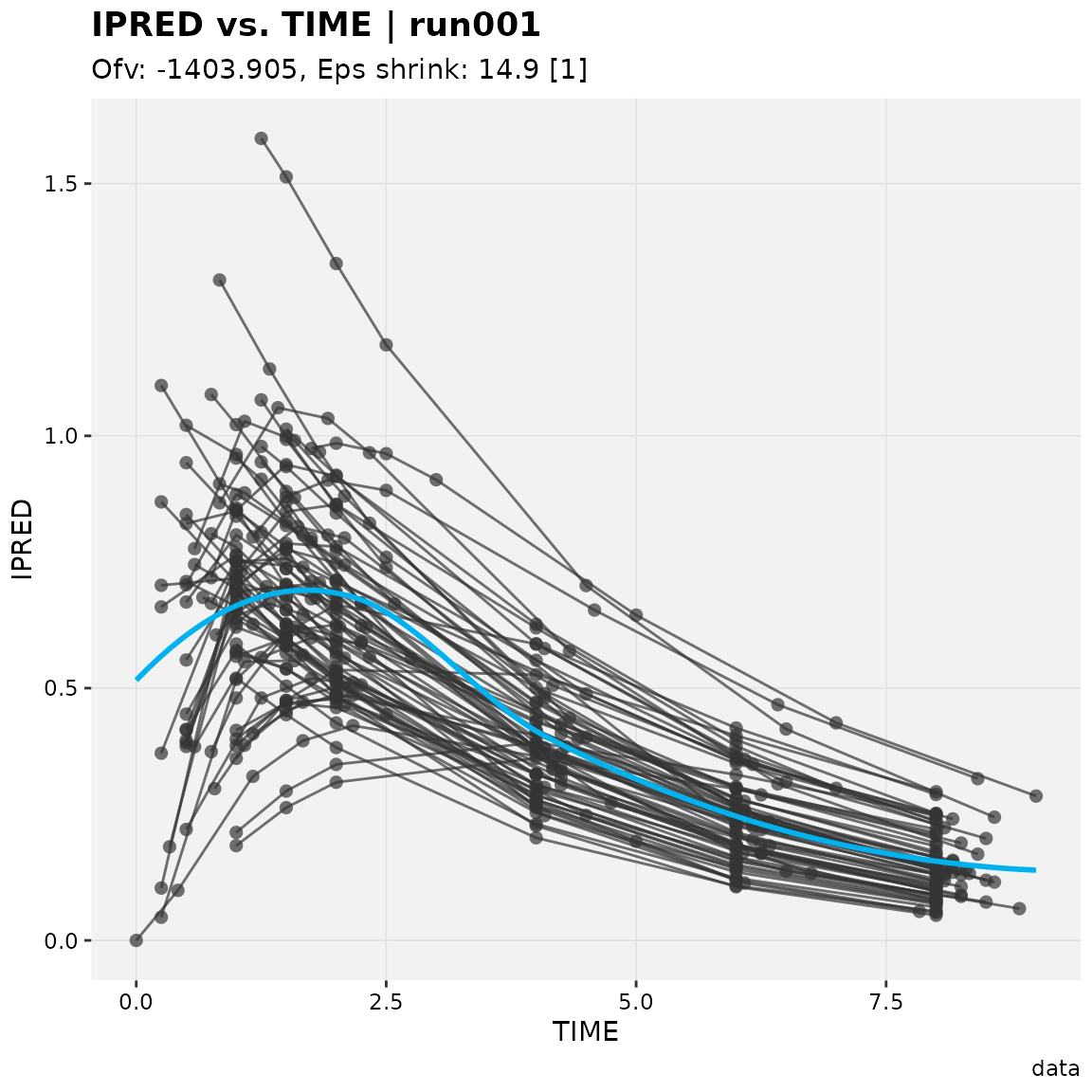pred_vs_idv(xpdb_ex_pk)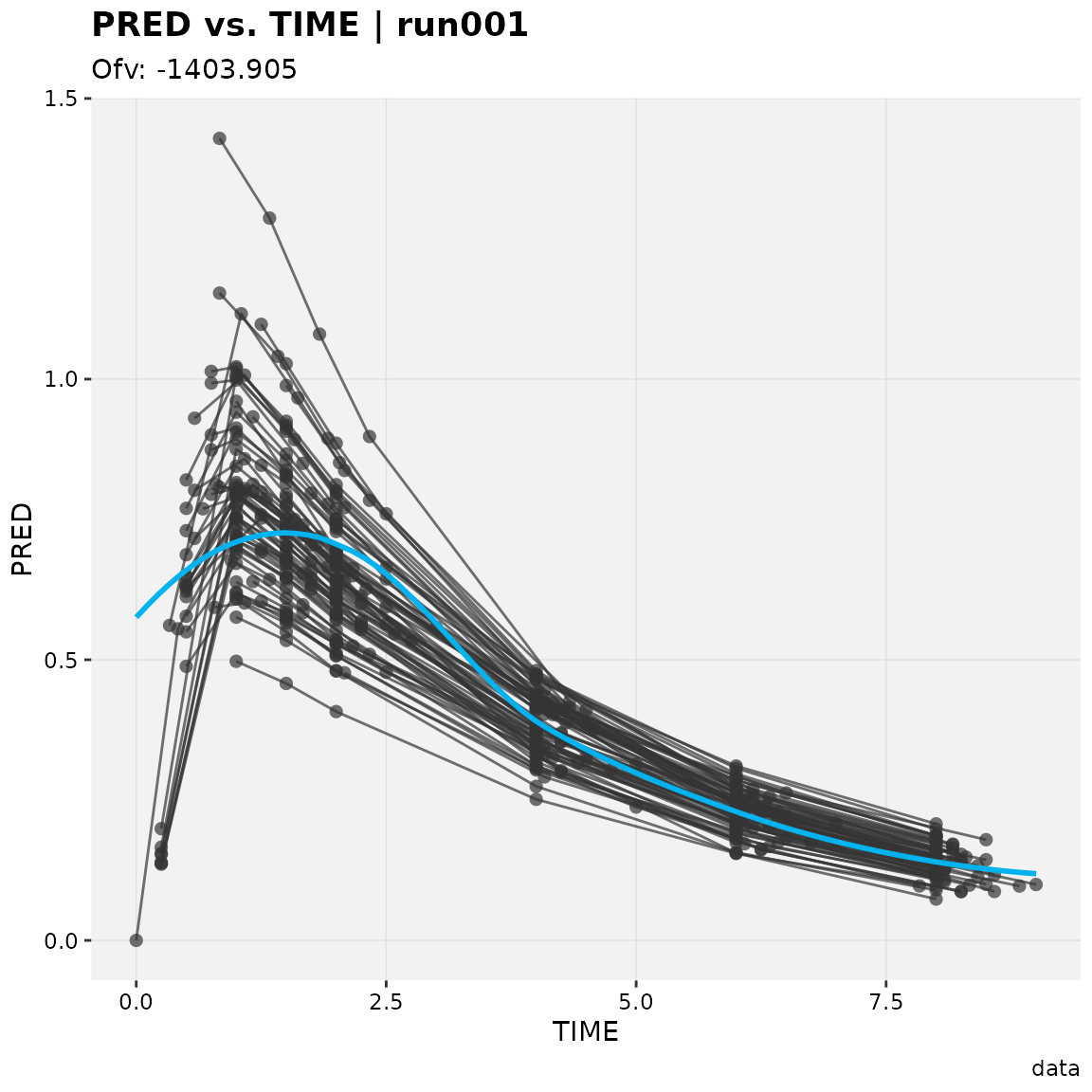dv_preds_vs_idv(xpdb_ex_pk)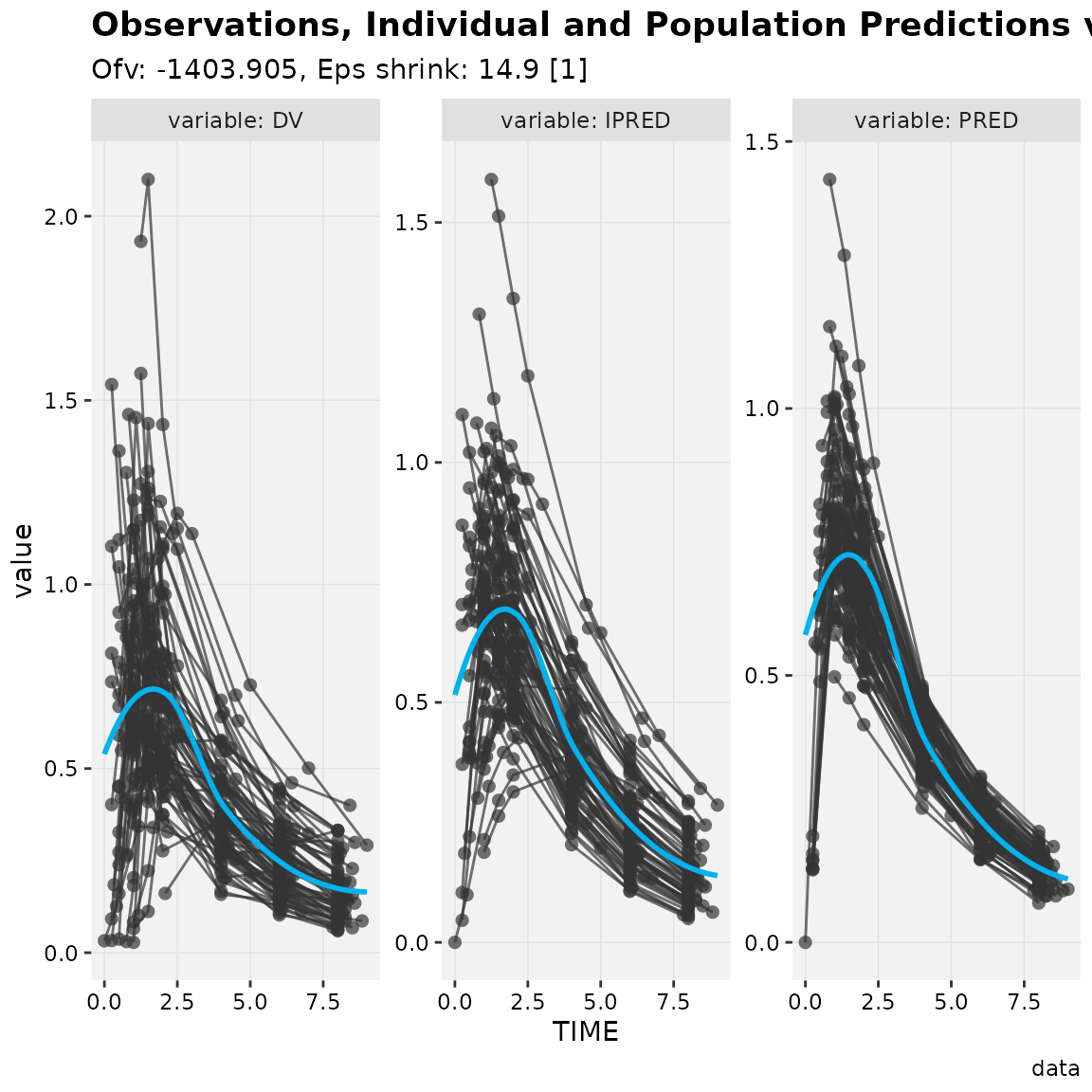### DV vs. PREDs plots

dv_vs_pred(xpdb_ex_pk)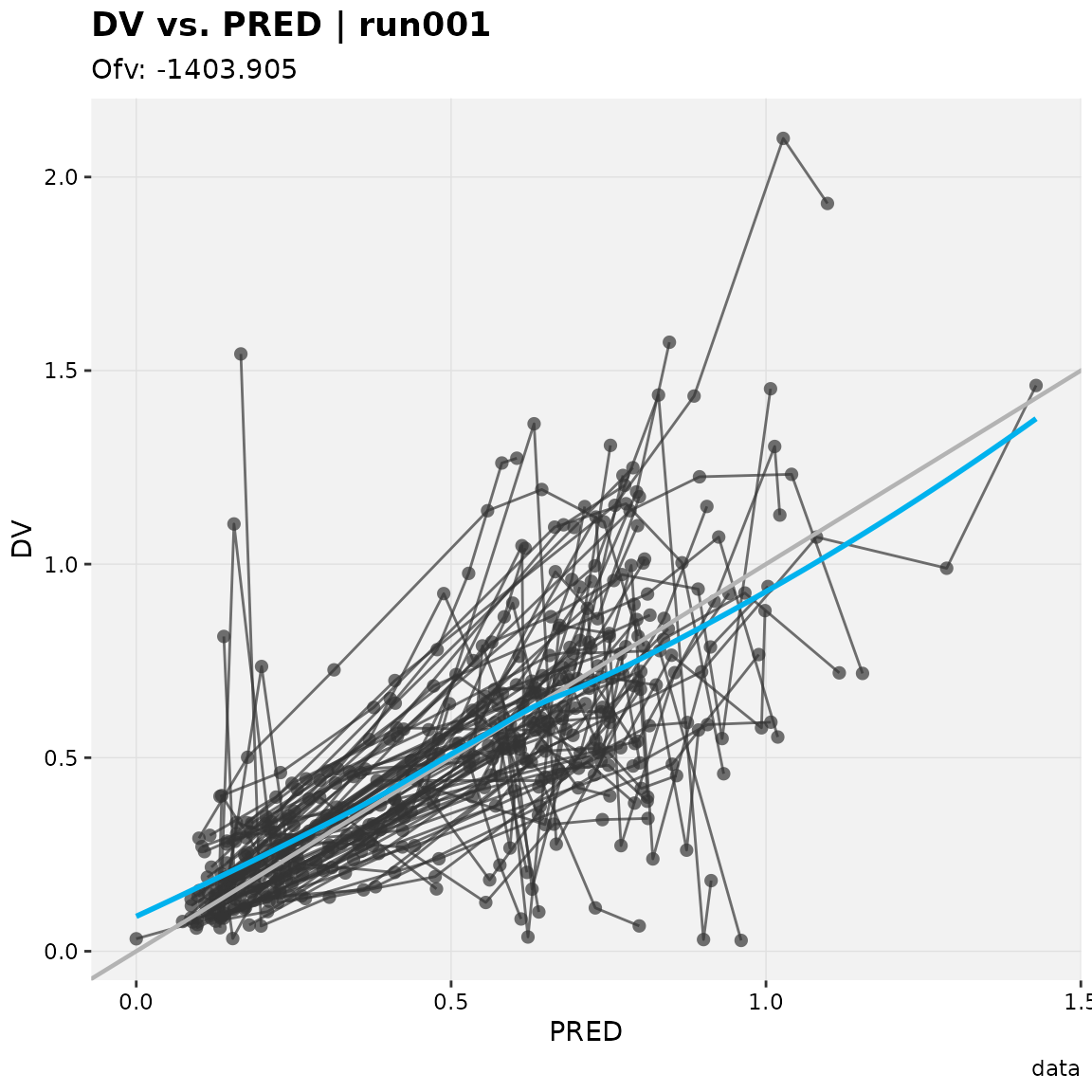dv_vs_ipred(xpdb_ex_pk)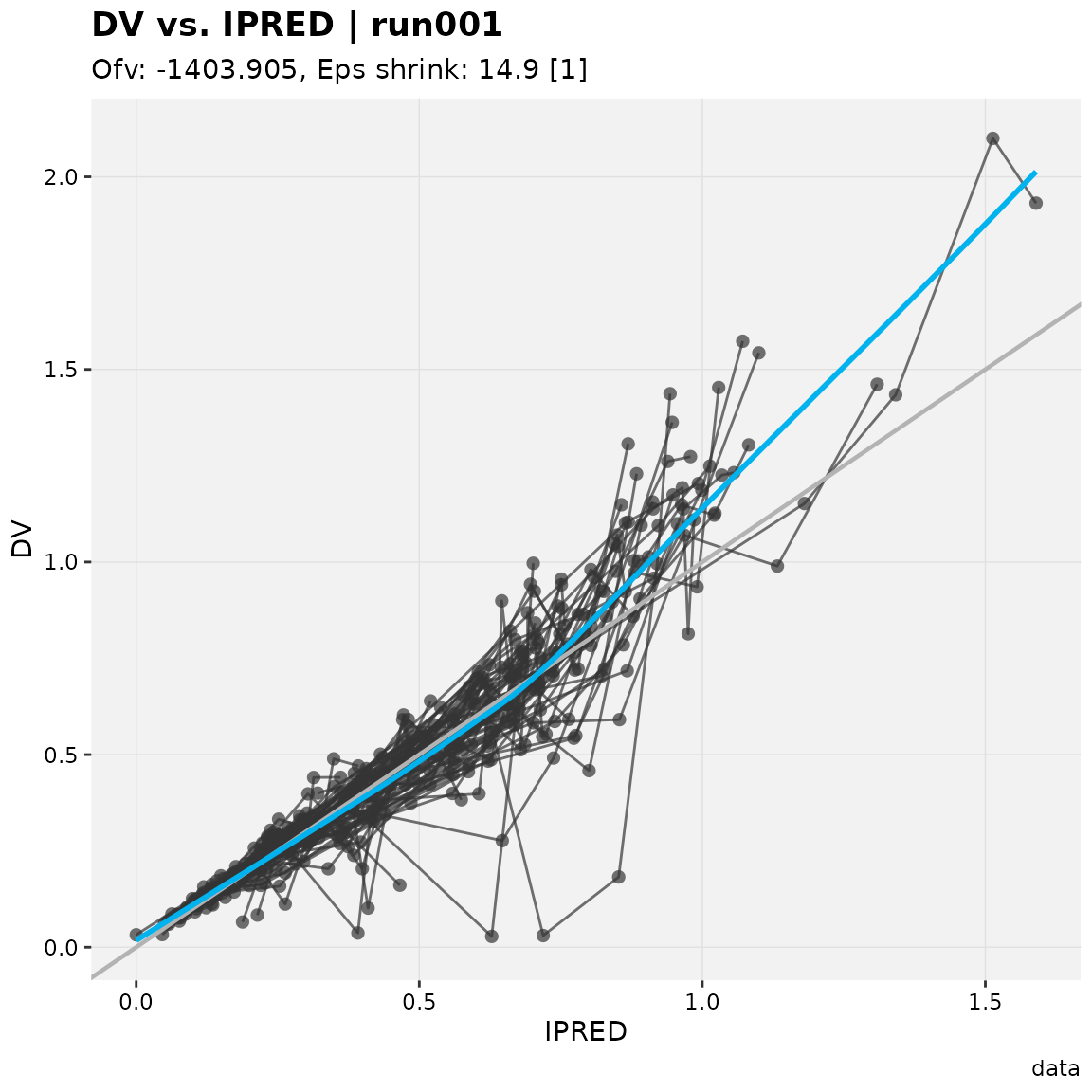### Residual plots

res_vs_idv(xpdb_ex_pk, res = 'CWRES')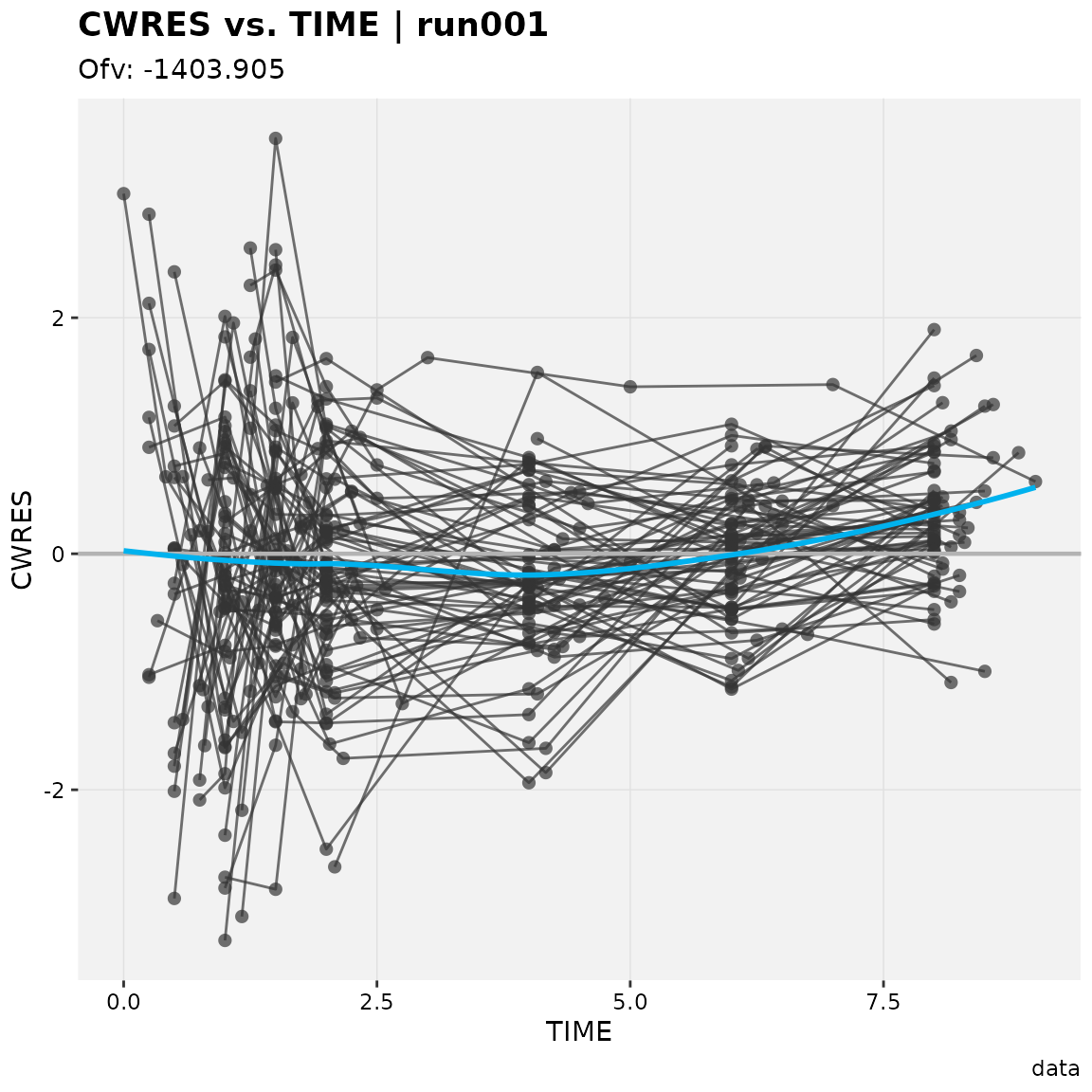res_vs_pred(xpdb_ex_pk, res = 'CWRES')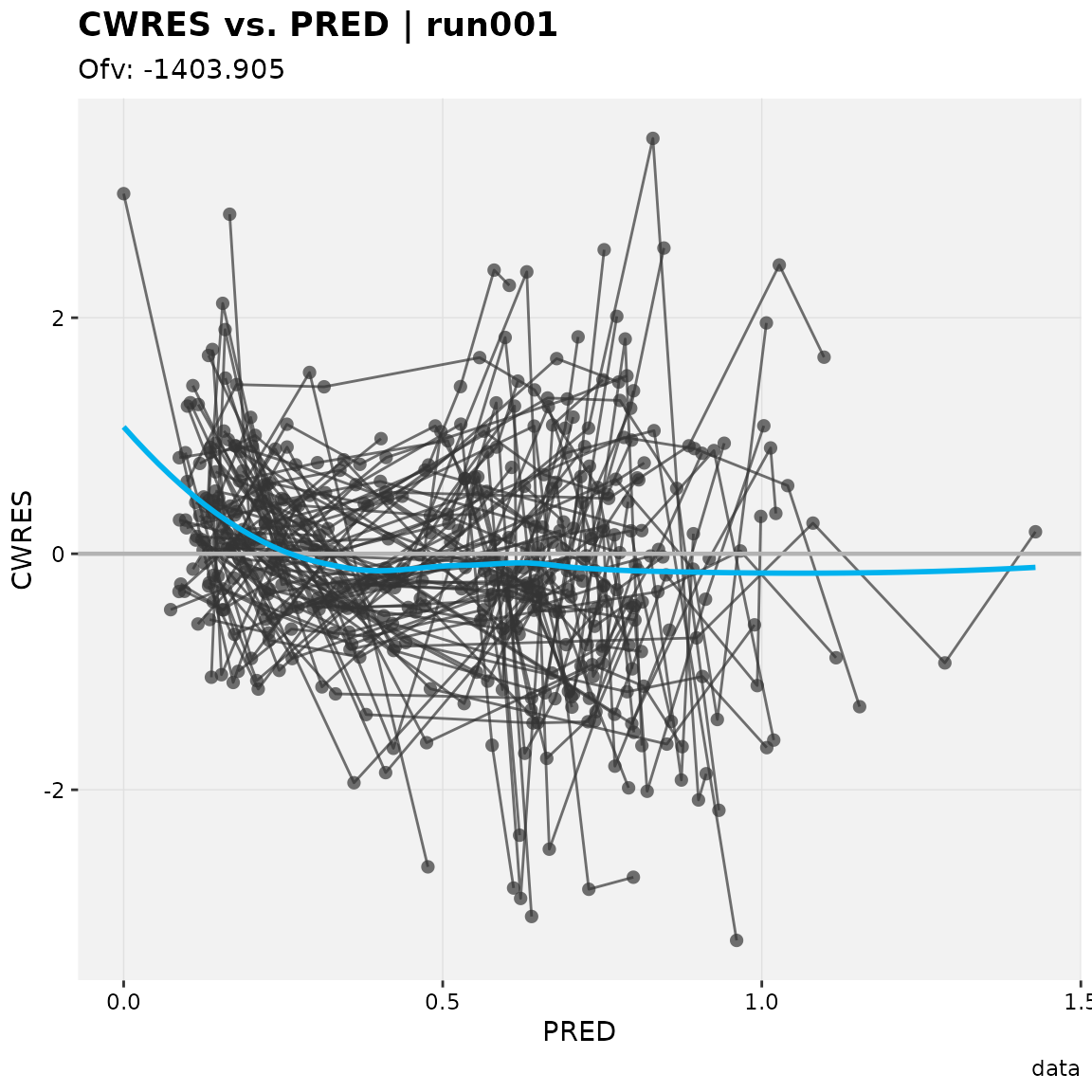absval_res_vs_idv(xpdb_ex_pk, res = 'CWRES')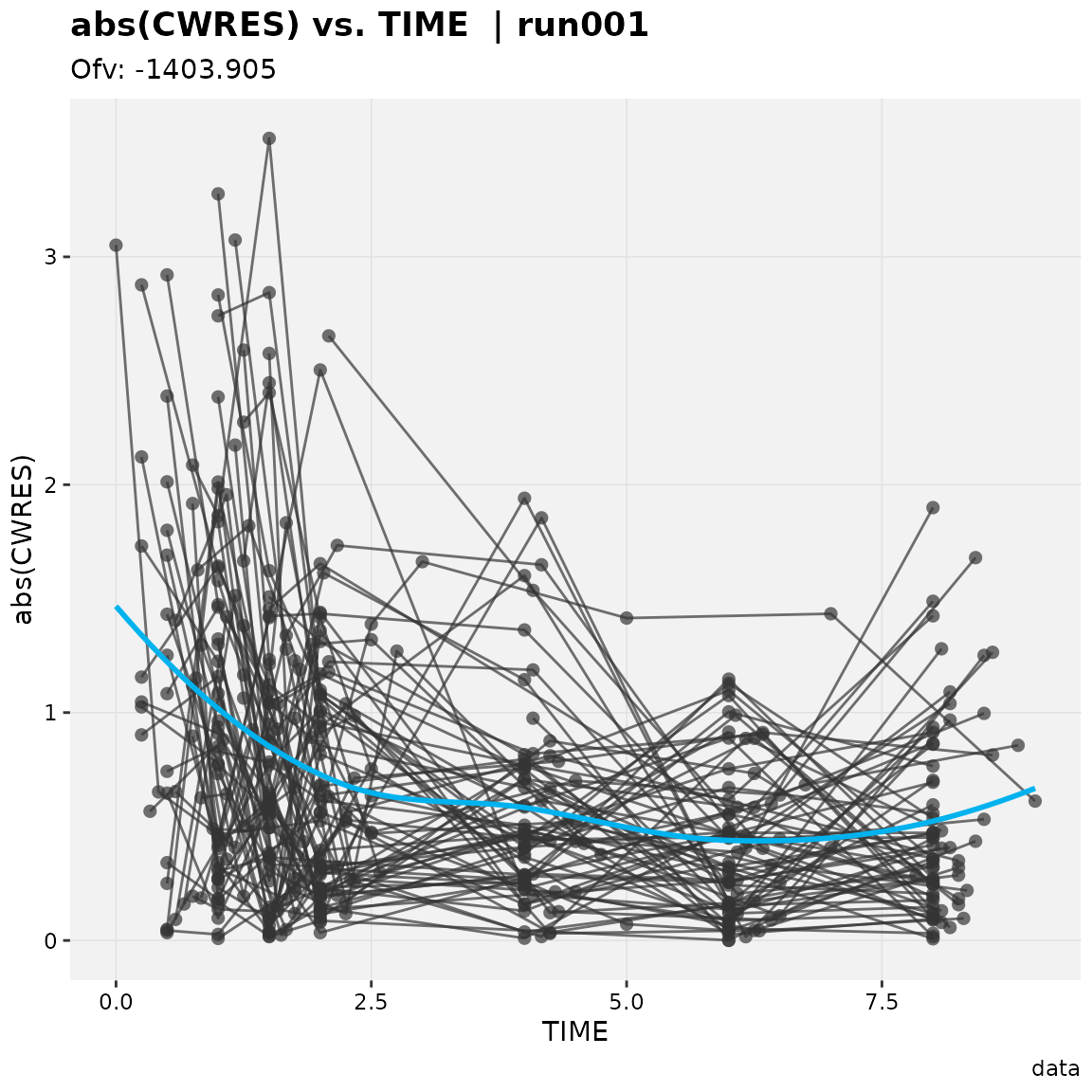absval_res_vs_pred(xpdb_ex_pk, res = 'CWRES')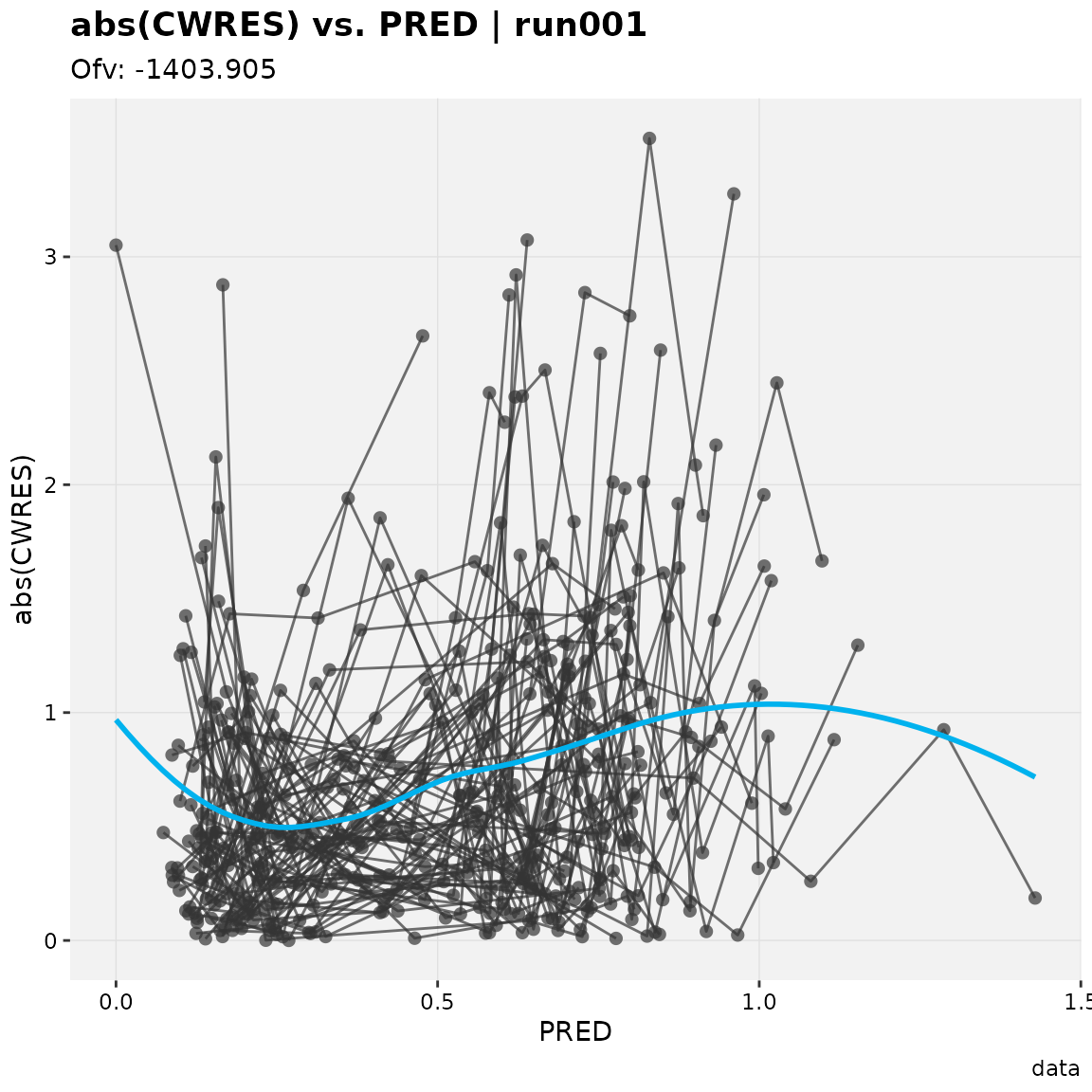## Minimization plots

prm_vs_iteration(xpdb_ex_pk, labeller = 'label_value')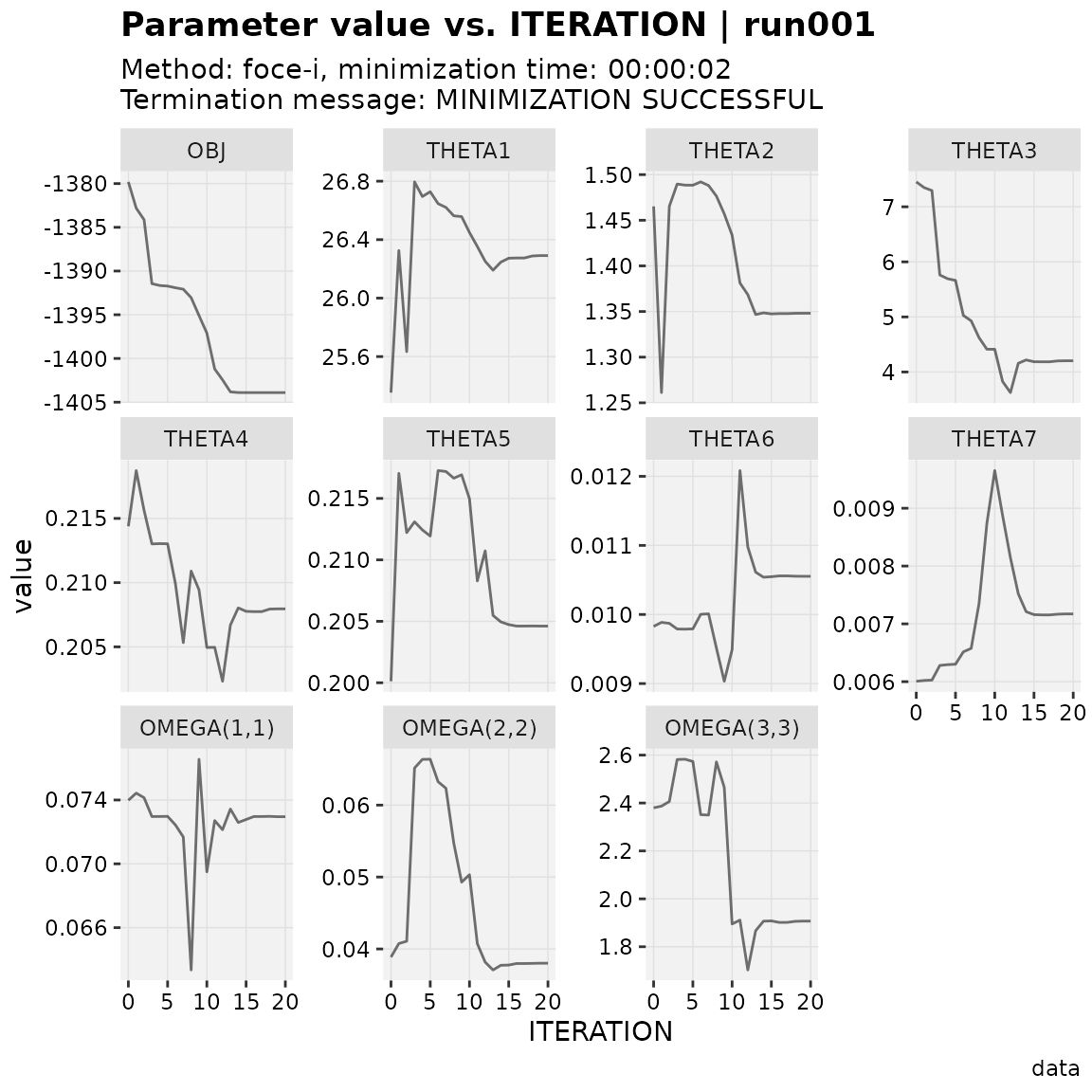grd_vs_iteration(xpdb_ex_pk, labeller = 'label_value')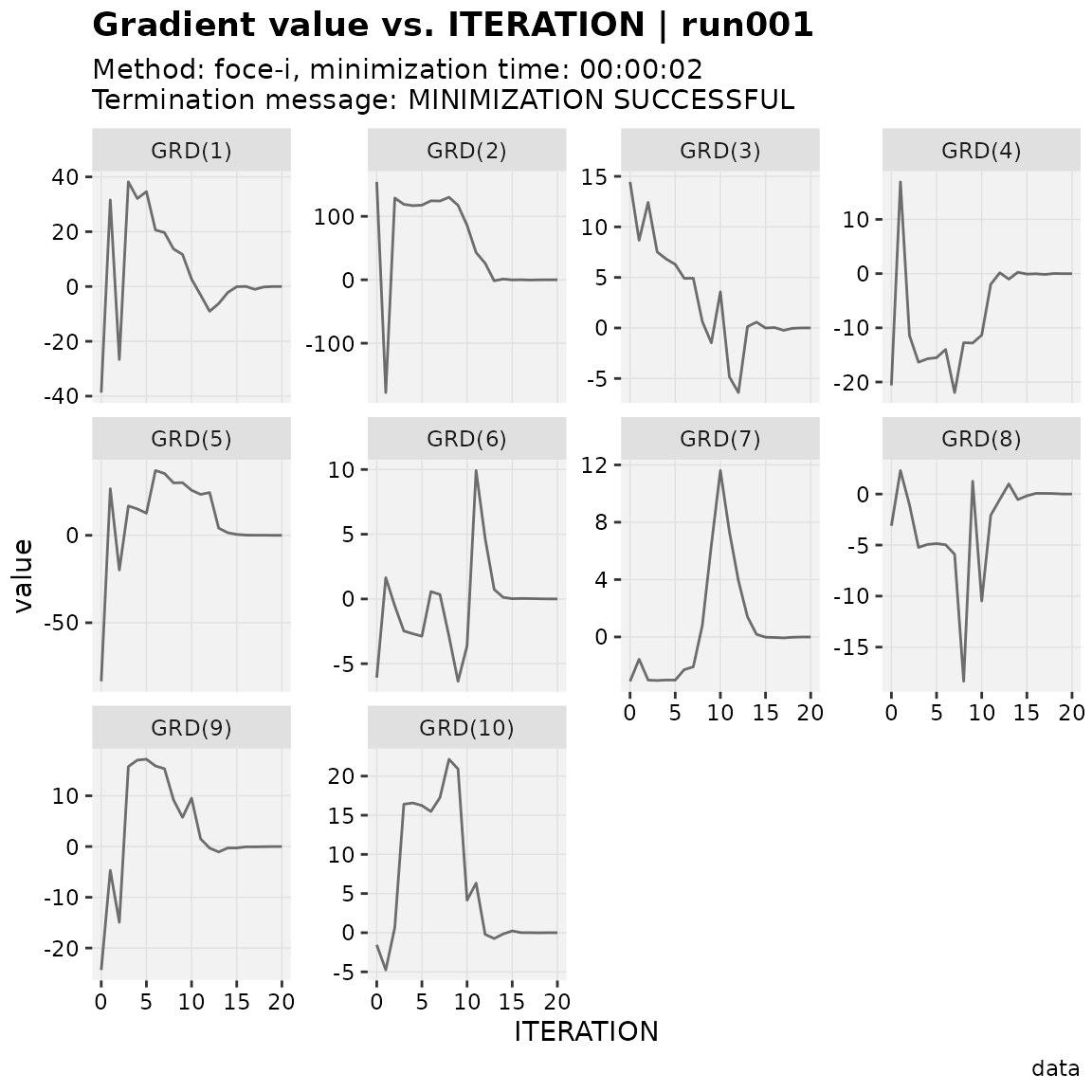## Individual plots

ind_plots(xpdb_ex_pk, page = 1)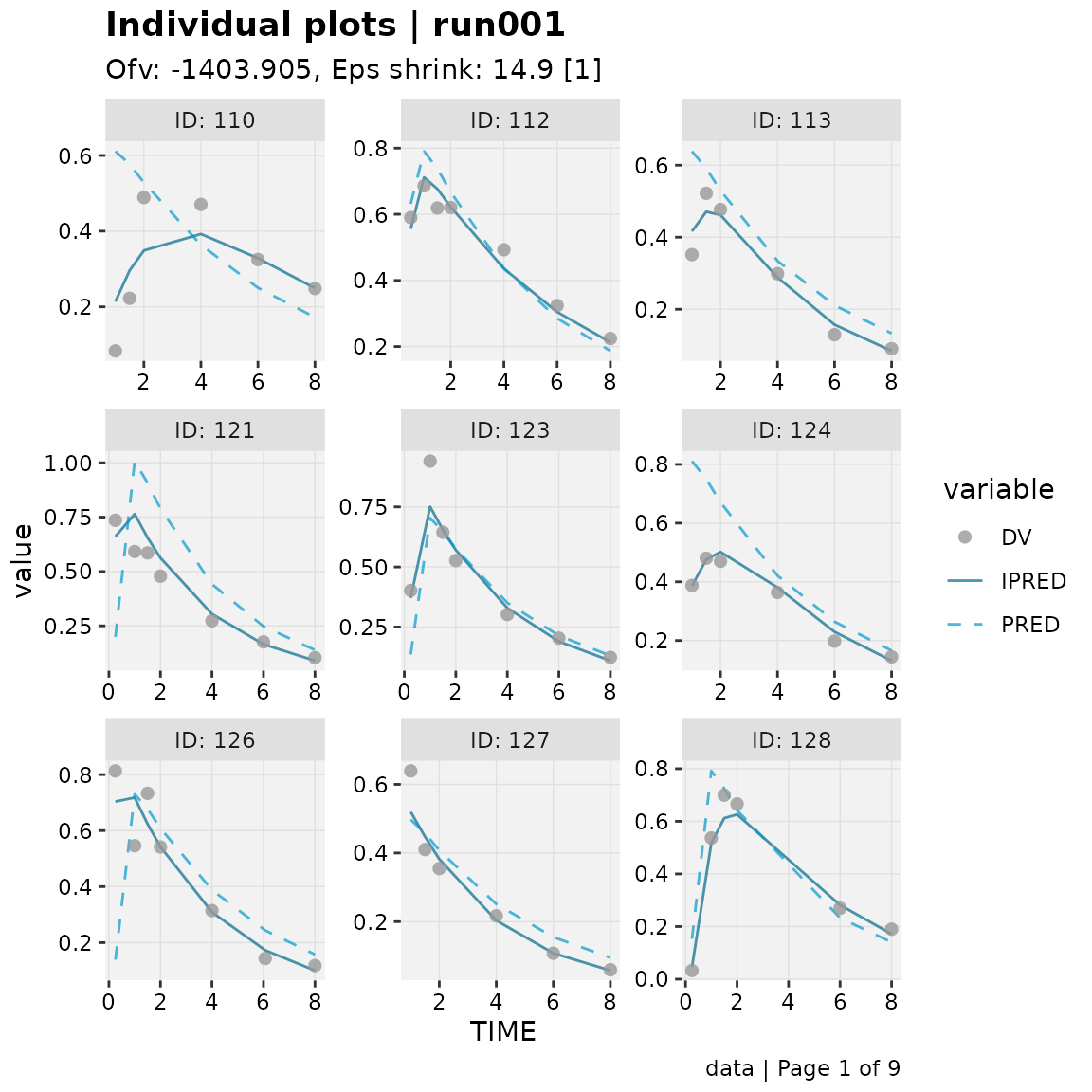## Distributions

### Histograms and density plots

prm_distrib(xpdb_ex_pk)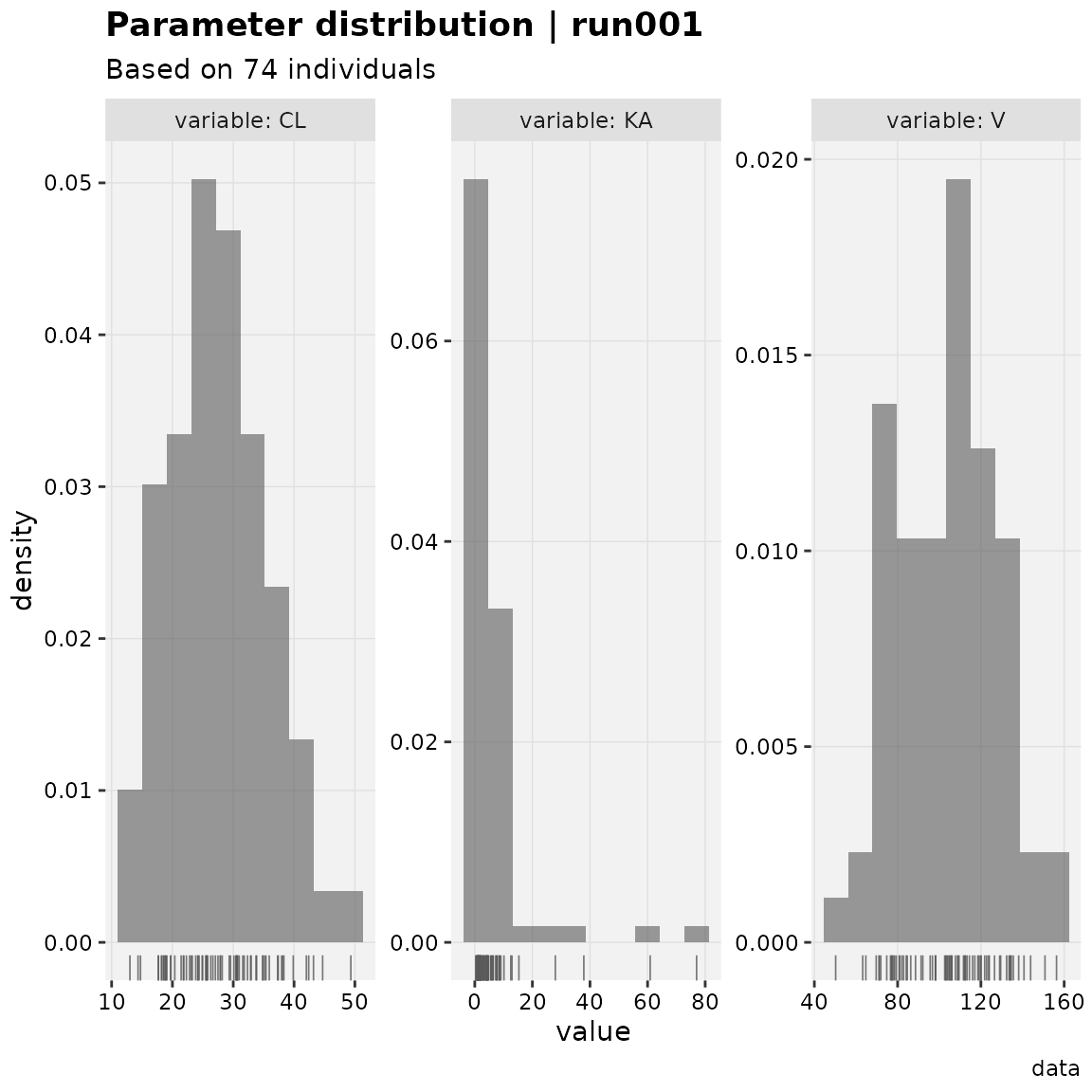eta_distrib(xpdb_ex_pk)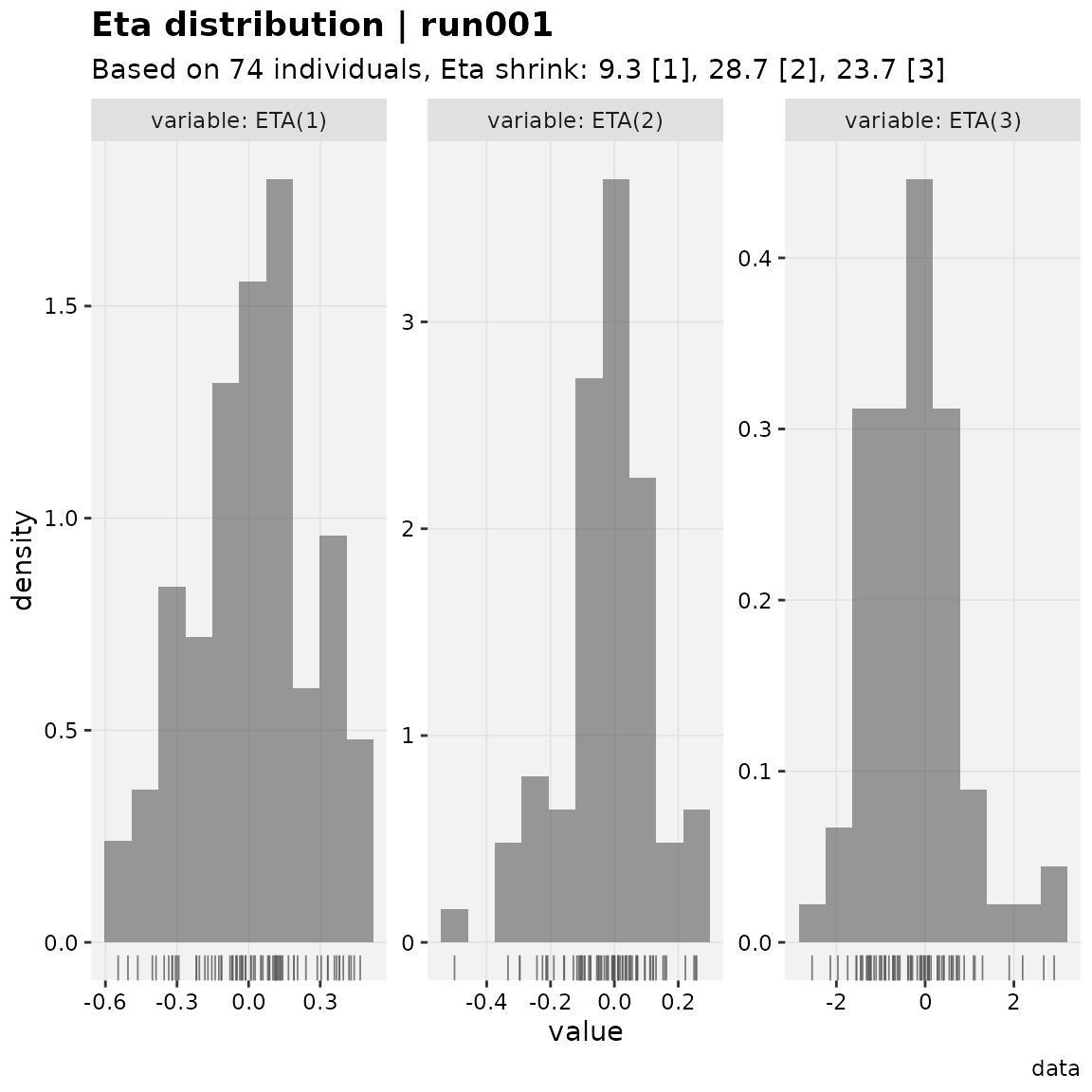res_distrib(xpdb_ex_pk)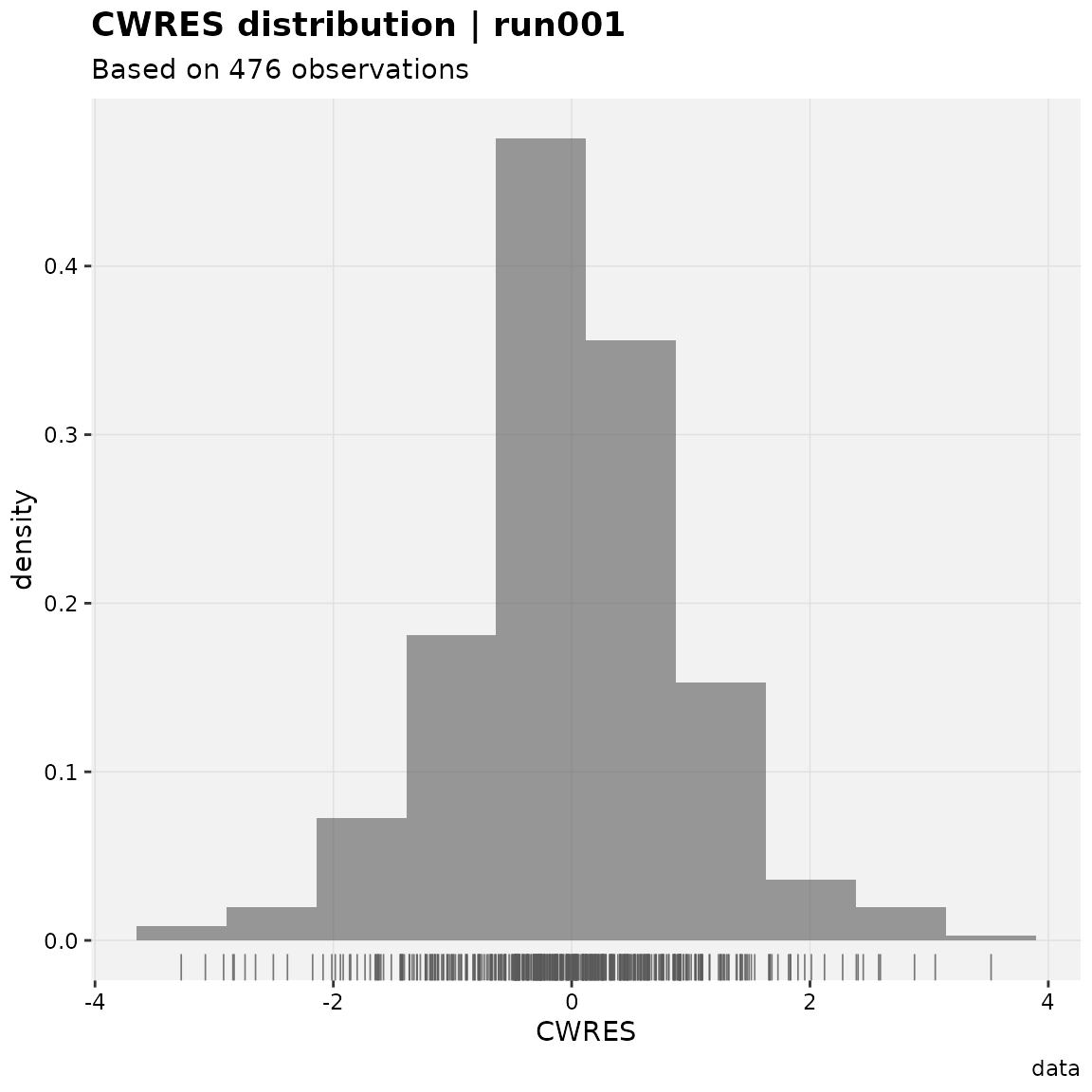cov_distrib(xpdb_ex_pk)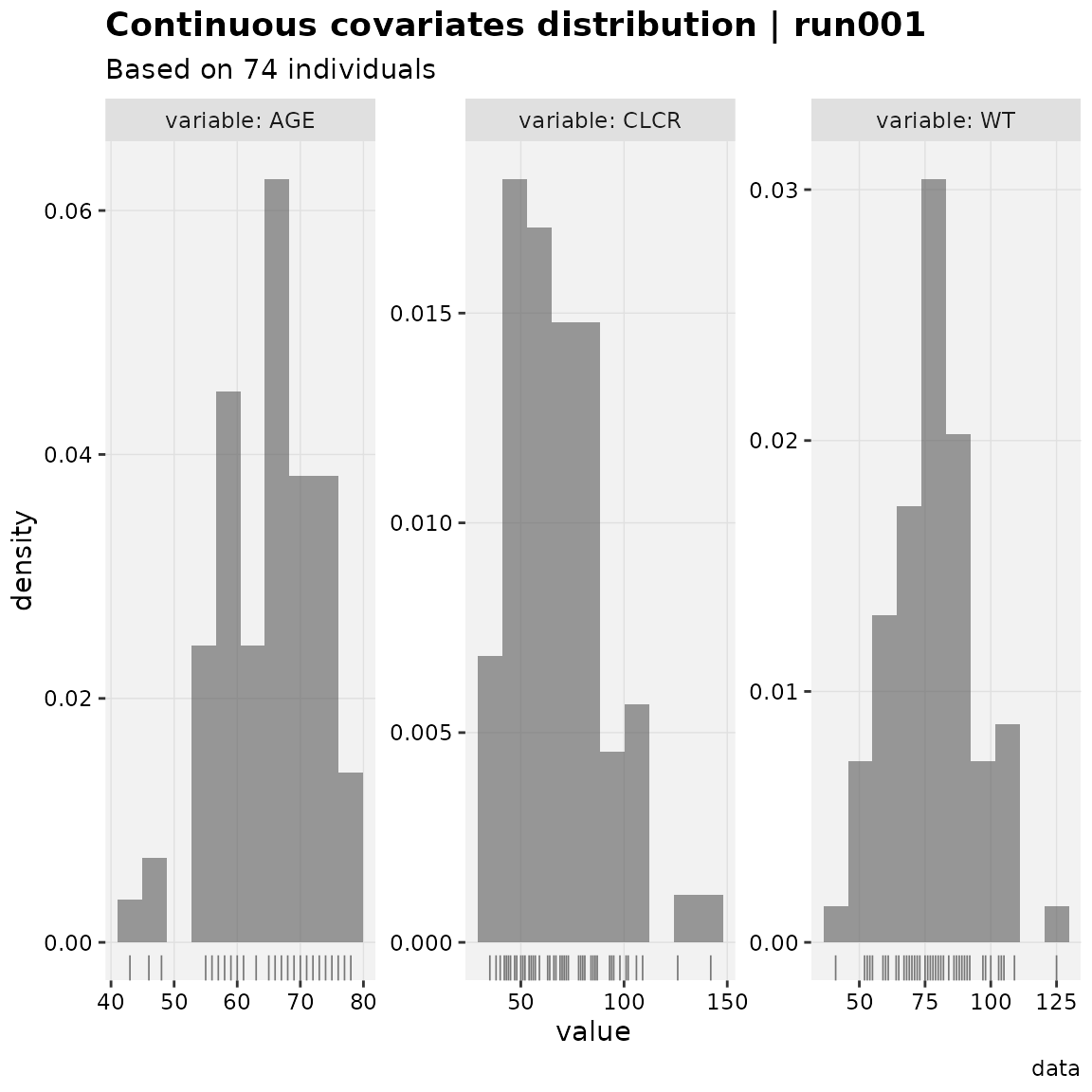### QQ plots

prm_qq(xpdb_ex_pk)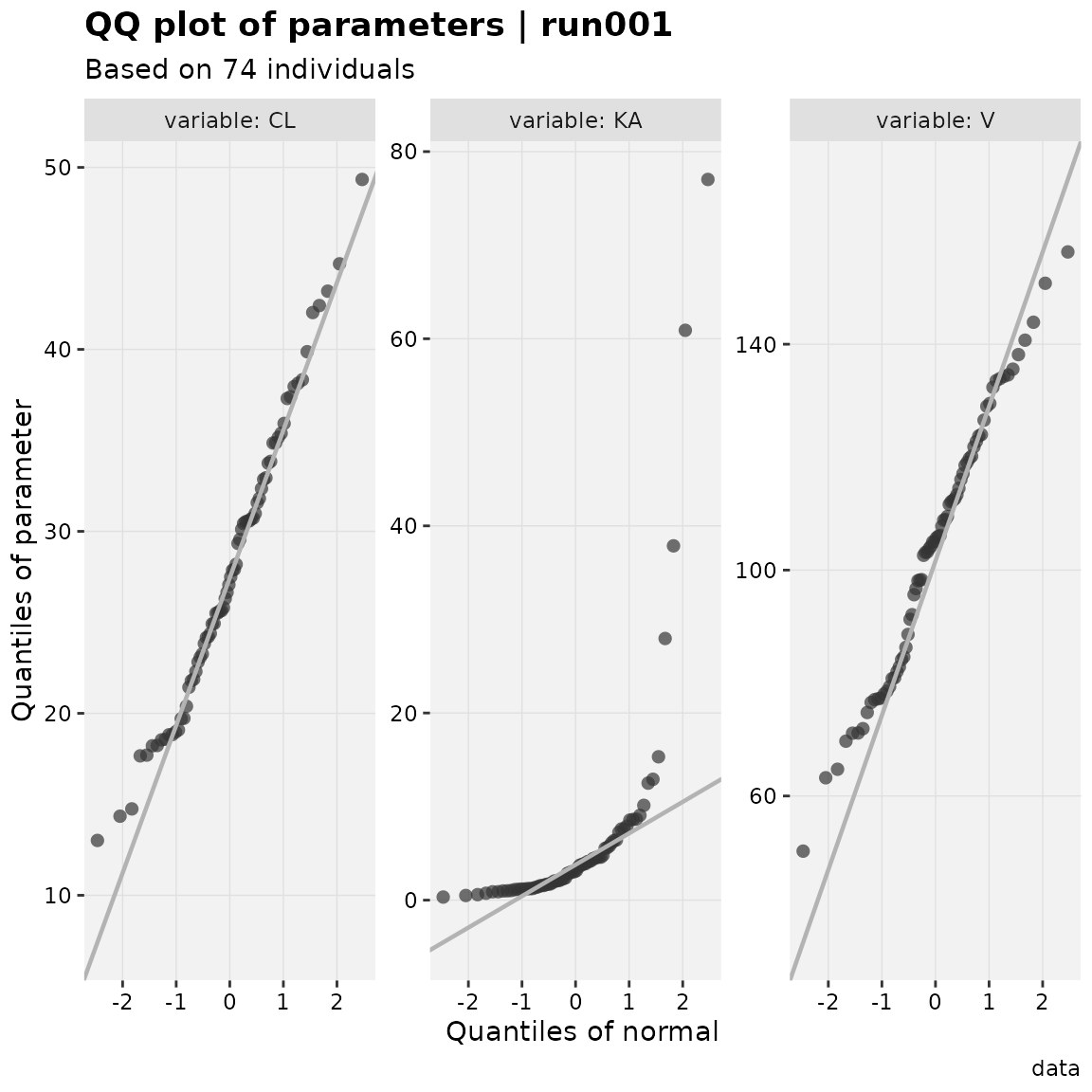eta_qq(xpdb_ex_pk)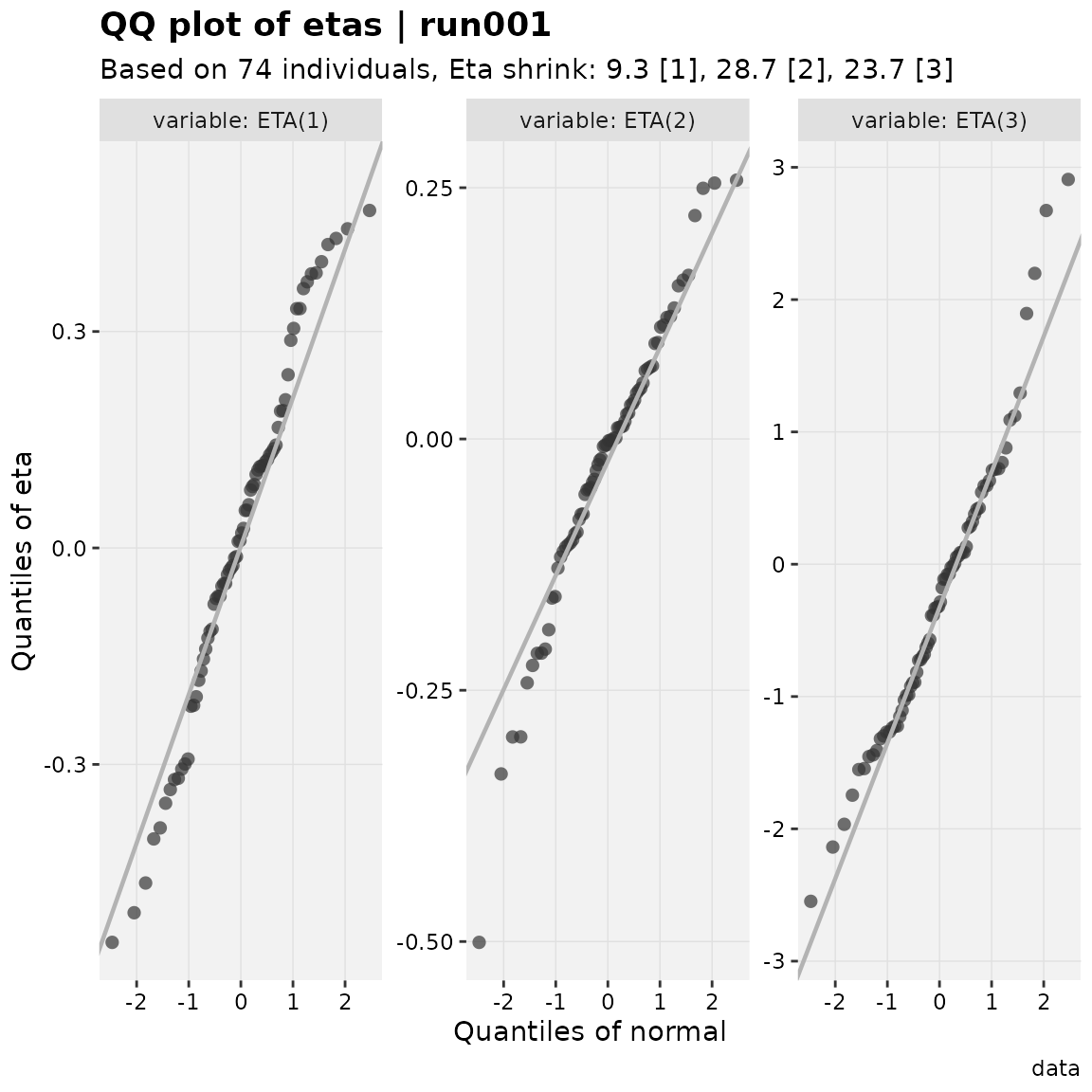res_qq(xpdb_ex_pk)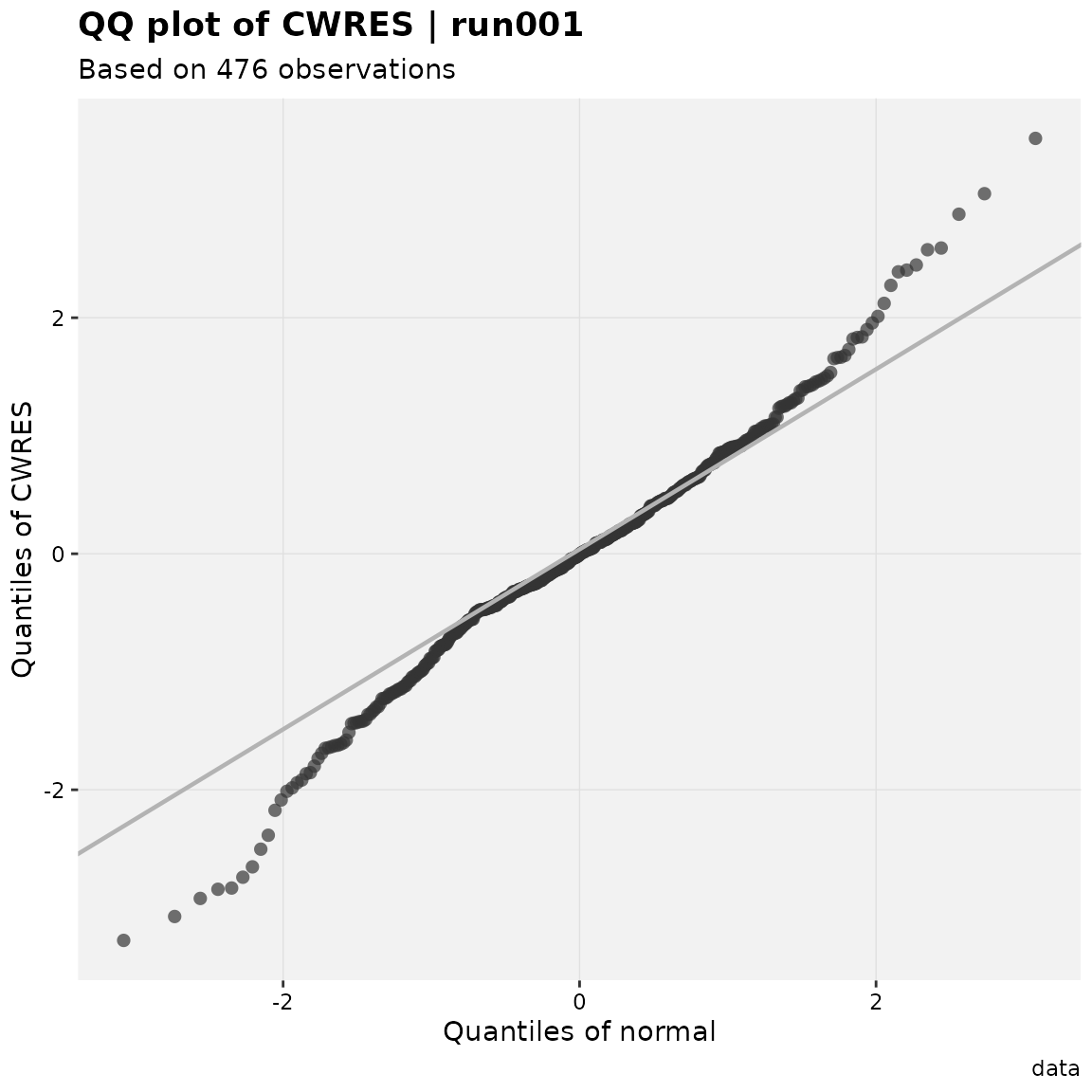cov_qq(xpdb_ex_pk)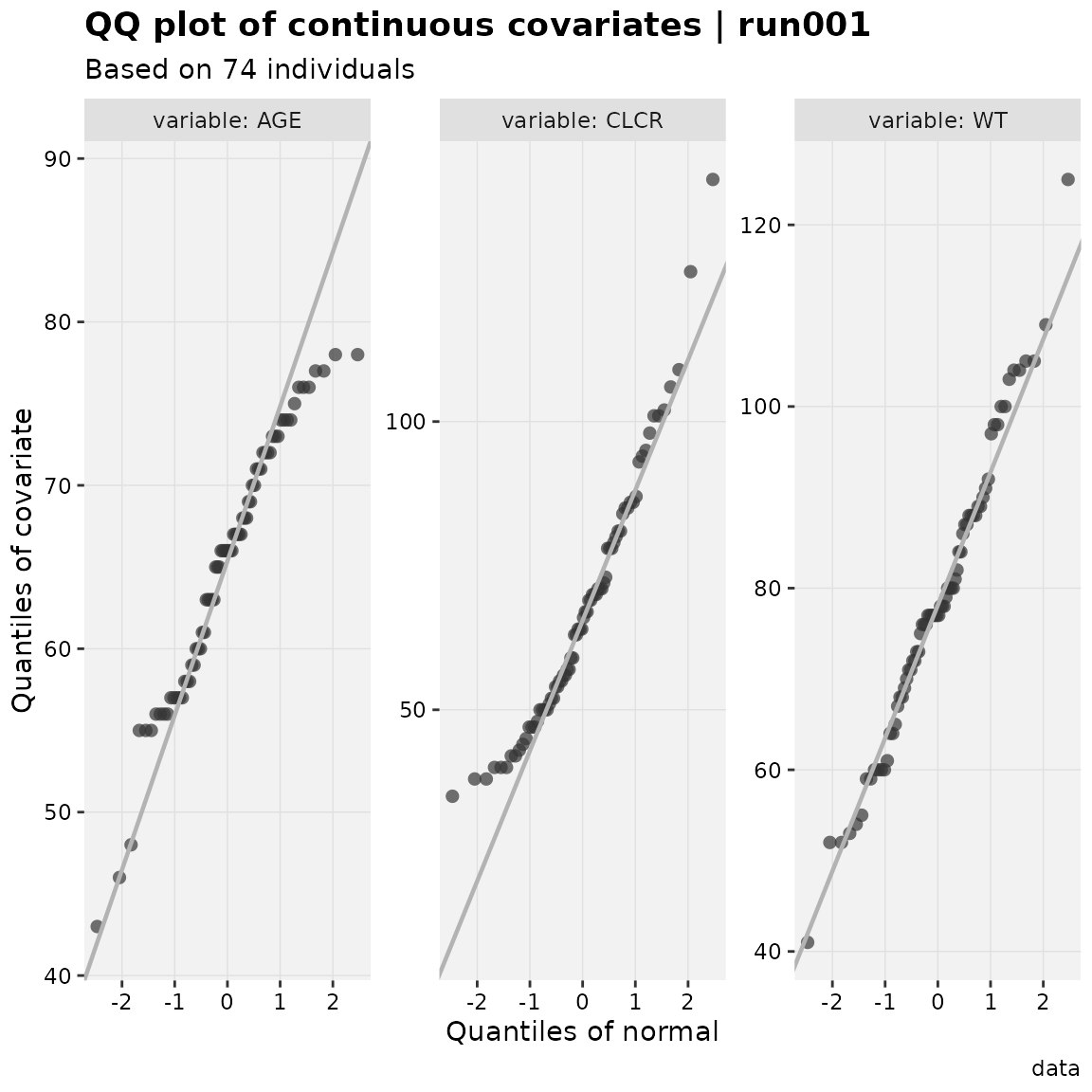## Kinetic plots

### Compartment’s amount

amt_vs_idv(xpdb_ex_pk)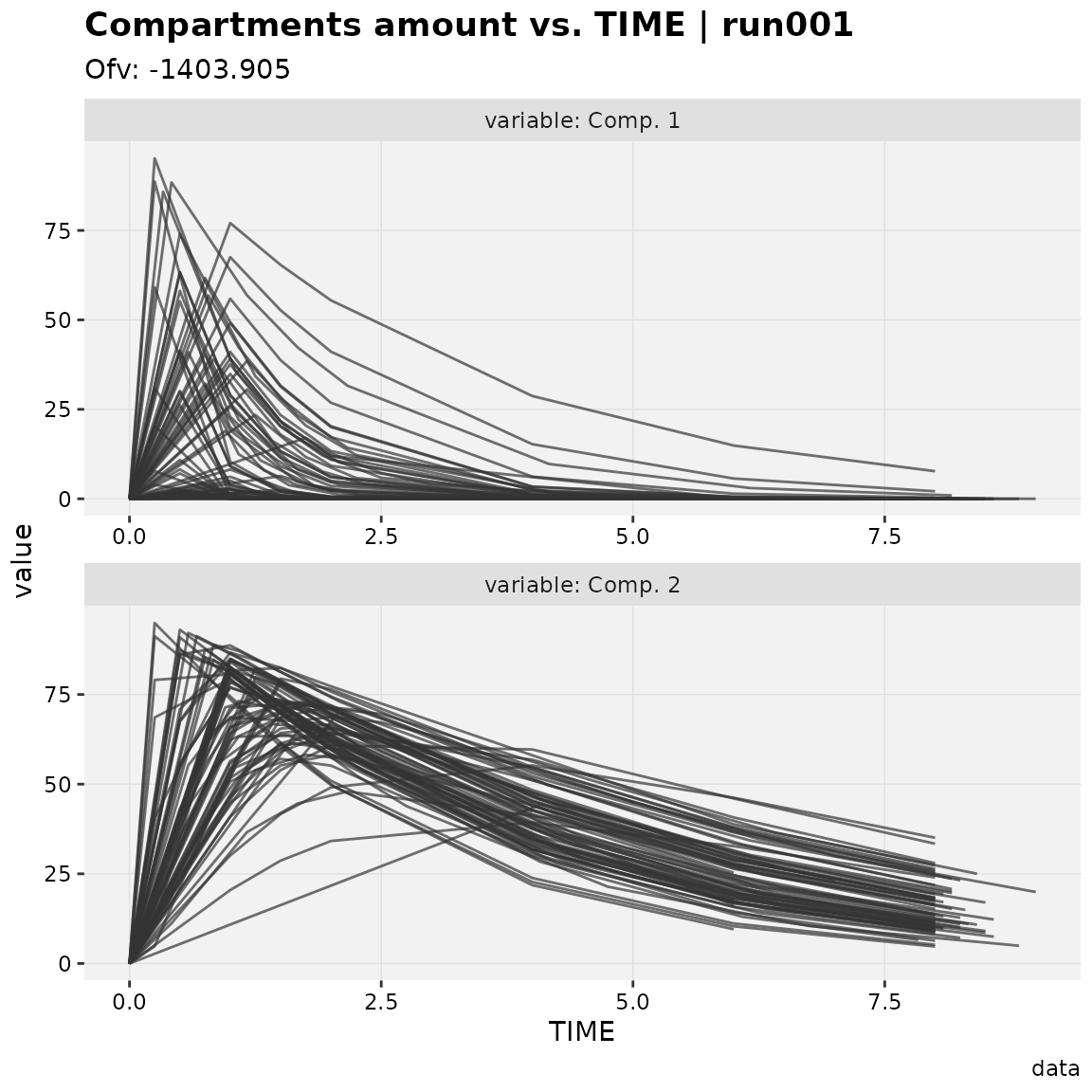## Visual predictive checks (VPC)

### Continuous vpc

xpdb_ex_pk %>%
vpc_data(opt = vpc_opt(n_bins = 7)) %>%
vpc()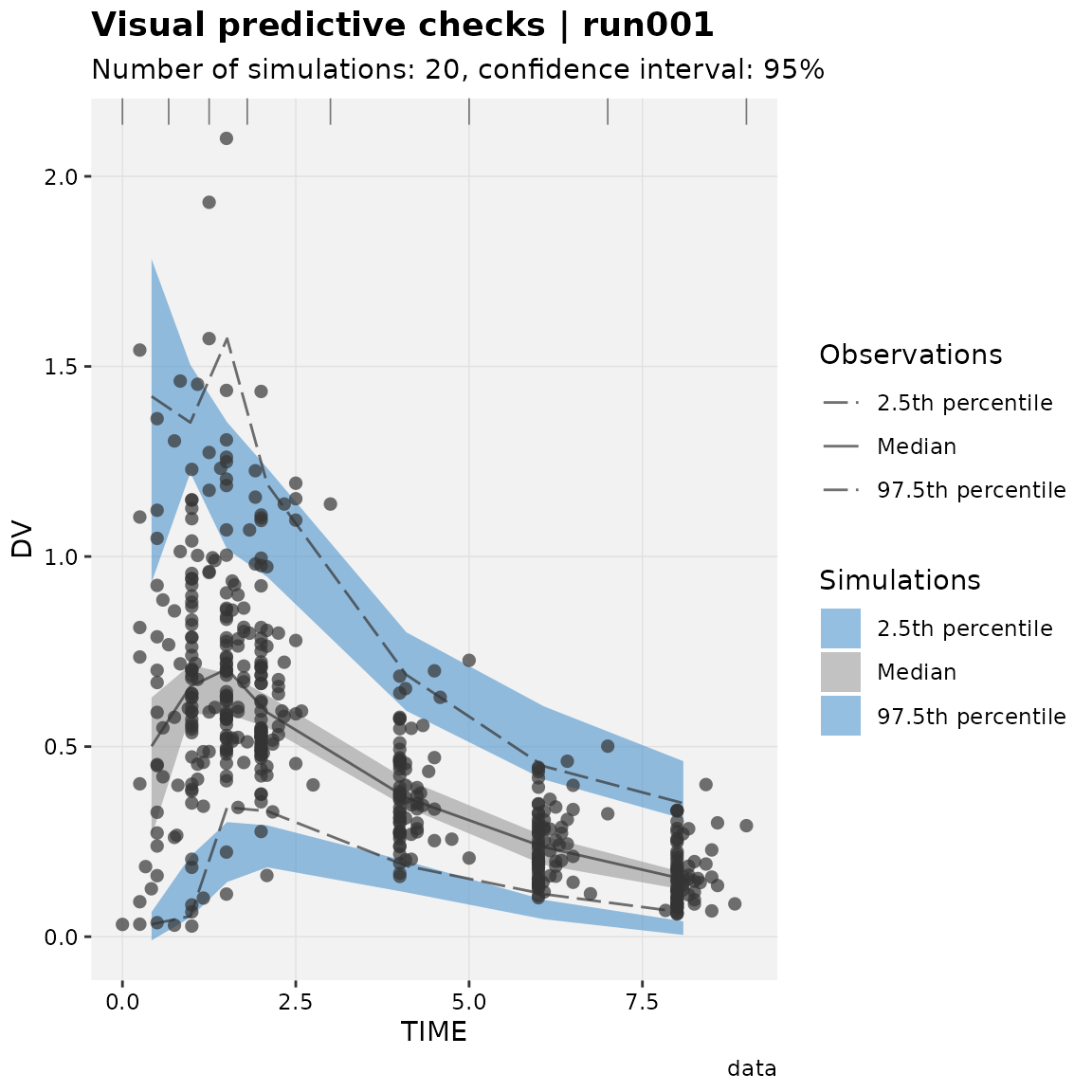### Censored vpc

xpdb_ex_pk %>%
vpc_data(vpc_type = 'censored', opt = vpc_opt(lloq = 1, n_bins = 7)) %>%
vpc()
Warning: Points (type = 'p') can only be added with continuous VPC.### Categorical vpc

xpdb_ex_pk %>%
vpc_data(vpc_type = 'categorical') %>%
vpc()